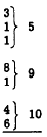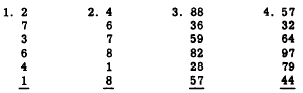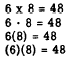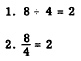Mental CalculationCustom SearchMental Calculation Mental regrouping can be used to avoid the necessity of writing down some of the steps, or of rewriting in columns, when groups of one-digit or two-digit numbers are to be added or subtracted. One of the most common devices for rapid addition is recognition of groups of digits whose sum is 10. For example, in the following problem two "ten groups" have been marked with braces:To add this column as grouped, you would say to yourself, "7, 17, 22, 32." The thought should be just the successive totals as shown above and not such cumbersome steps as "7 + 10, 17, + 5, 22, + 10, 32." When successive digits appear in a column and their sum is less than 10, it is often convenient to think of them, too, as a sum rather than separately. Thus, if adding a column in which the sum of two successive digits is 10 or less, group them as follows:The thought process here might be, as shown by the grouping, "5, 14, 24." Multiplication may be indicated by a multiplication sign (x) between two numbers, a dot  Practice problems. Add the following columns from the top down, as in the preceding example:Answers, showing successive mental steps: 1. 2, 12, 22, 23 - - Final answer, 23 2. 10, 17, 26, 34 - - Final answer, 34 3. Units column: 14, 23, 33, 40 - - Write down 0, carry 4.     Tens column: 12, 20, 30, 35 - - Final answer, 350. 4. Units column: 9, 20, 29, 33 - - Write down 3, carry 3.     Tens column: 8, 17, 26, 37 - - Final answer, 373. SUBTRACTION.-In an .example such as 73 - 46, the conventional approach is to place 46 under 73 and subtract units from units and tens from tens, and write only the difference without the intermediate steps. To do this, the best method is to begin at the left. Thus, in the example 73 - 46, we take 40 from 73 and then take 6 from the result. This is done mentally, however, and the thought would be "73, 33, 27," or "33, 27." In the example 84 - 21 the thought is "64,63" and in the example 64 - 39 the thought is "34, 25." Practice problems. Mentally subtract and write only the difference: 1. 47 - 24  2. 69 - 38  3. 87 - 58  4. 86 - 73 5. 82 - 41 6. 30 - 12 Answers, showing successive mental steps: 1. 27, 23 - - Final answer, 23 2. 39, 31 - - Final answer, 31 3. 37, 29 - - Final answer, 29 4. 16, 13 - - Final answer, 13 5. 42, 41 - - Final answer, 41 6. 20, 18 - - Final answer, 18 MULTIPLICATION AND DIVISION between two numbers, or parentheses around one or both of the numbers to be multiplied. The following examples illustrate these methods:Notice that when a dot is used to indicate multiplication, it is distinguished from a decimal point or a period by being placed above the line of writing, as in example 2, whereas a period or decimal point appears on the line. Notice also that when parentheses are used to indicate multiplication, the numbers to be multiplied are spaced closer together than they are when the dot or x is used. In each of the four examples just given, 6 is the MULTIPLIER and 8 is the MULTIPLICAND. Both the 6 and the 8 are FACTORS, and the more modern texts refer to them this way. The "answer" in a multiplication problem is the PRODUCT; in the examples just given, the product is 48. Division usually is indicated either by a division sign (+) or by placing one number over another number with a line between the numbers, as in the following examples:The number 8 is the DIVIDEND, 4 is the DIVISOR, and 2 is the QUOTIENT.Integrated Publishing, Inc. - A (SDVOSB) Service Disabled Veteran Owned Small Business